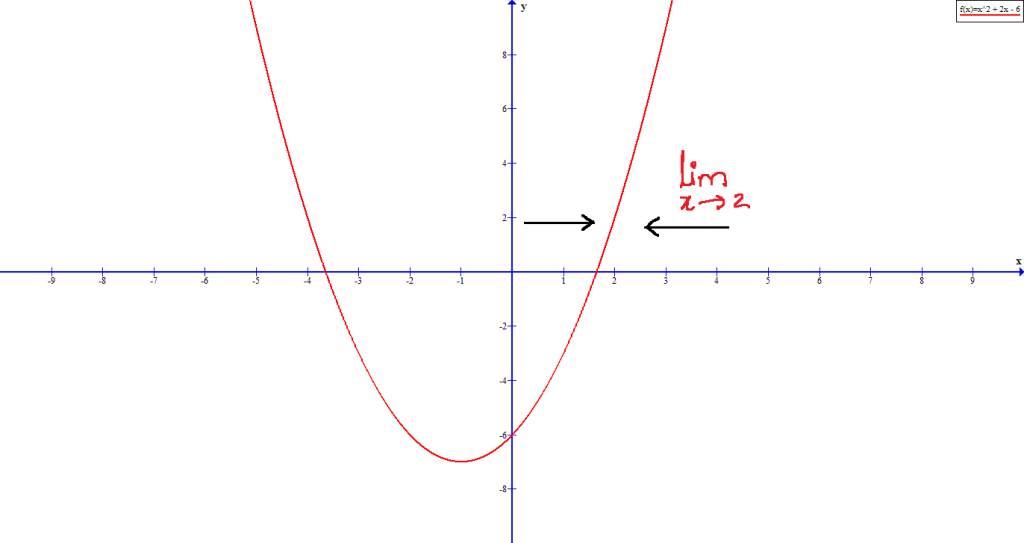# Understanding Limits and Continuity

Limits and continuity of function is where calculus topics starts, however, to know calculus you must complete all lessons of pre- calculus with examples.

Limit of a function is a certain value that a function approaches, whereas a function is said to be continuous when the function has no breaks or drawn without taking the pen away from the paper.

### How to understand a limit of a function?

Limit of a function is a certain value on x- axis that a function of x approaches from both the sides. When a function of x approaches to n (where n is an integer), then all the values of x are very close to n from both the side of the number line. For example, when function of x approaches to 2, then the the values near to the limit of a function from the left if 1.9, 1.99, 1.999 etc. and from the right is 2.1, 2.01, 2.001 etc.

### Limit with an example

Let us say$f(x) = x^{2} + 2x - 6$ is a function whose limit is 2, then, the limit of a function can be written as$\displaystyle \lim_{x \to 2}$. This can be showed in the form of a graph.Figure 1 – Limit example, the value of x approaches 2 from left and right side but never equal to 2.

While finding the limit of a function, what should we observe?

Finding the limit of a function is all about observing the particular value a function approaches from left and right. From figure 1, it can be observed that both the right hand limit and left hand limit of a function approaches to the same number. In the above graph, the limit approaches from both right and left to 2.$\displaystyle \lim_{x \to c^-}$ means that x approaches c from left and reach all numbers smaller than c.
Similarly,$\displaystyle \lim_{x \to c^-}$ means that x approaches c from right and reach all numbers greater than c.

Note: the value of x is never equal to c.

### Continuity of a function

The limits applies to a continuous function. If the function is continuous, then a limit exists, otherwise not.

What is continuity of a given function?

A function is said to be continuous, when graph for the given function shows no breaks or discontinuity at a point or at least in a given interval.

How to find whether a function is continuous?

Continuity of a function can be examined in two ways.

• Continuity of a function at a given point
• Continuity over a given interval

For finding the continuity of is given function is, the given function should have no breaks at the neighbourhood to a given point.

How to determine the continuity of a function at a given point?

For checking the continuity of a function at a given point, then it must satisfy the following conditions viz.,

• The point at which continuity of a function is examined should be within the domain of the function.
• Both the left handed and right handed limit of the function approaching to the point should be equal.

### Summary

• Limit of a function is approached from both the sides.
• If the difference between the nearest value and the limit n is$h, h_{1}, h_{2}$ etc., then the values approaching from the left is$n - h, n - h_{1}, n - h_{2}$.
• In the same case, the values approaching from the right is$n + h, n + h_{1}, n + h_{2}$ .
• Continuity is defined as a function without any breaks or jumps.